Скачать презентацию Minimized compact automaton for clumps over degenerate patterns

• Количество слайдов: 17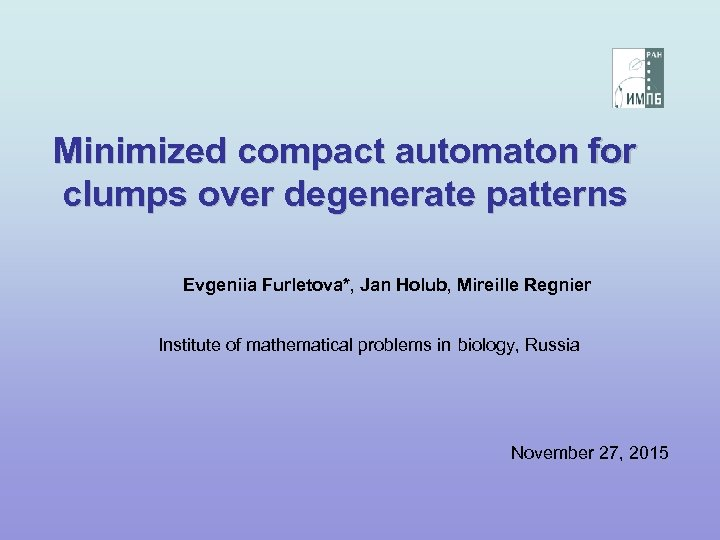Minimized compact automaton for clumps over degenerate patterns Evgeniia Furletova*, Jan Holub, Mireille Regnier Institute of mathematical problems in biology, Russia November 27, 2015Collaborators Mireille Regnier Ecole Polytechnique, INRIA, France Jan Holub Czech Technical University in Prague, Czech Republic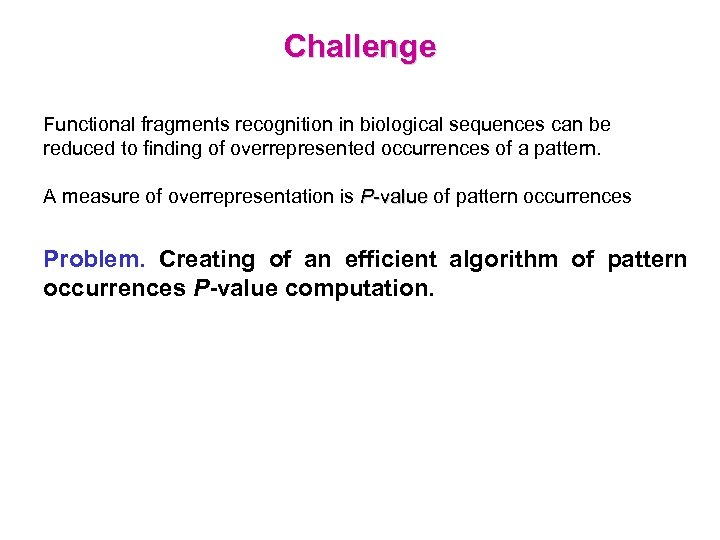Challenge Functional fragments recognition in biological sequences can be reduced to finding of overrepresented occurrences of a pattern. A measure of overrepresentation is P-value of pattern occurrences -value Problem. Creating of an efficient algorithm of pattern occurrences P-value computation.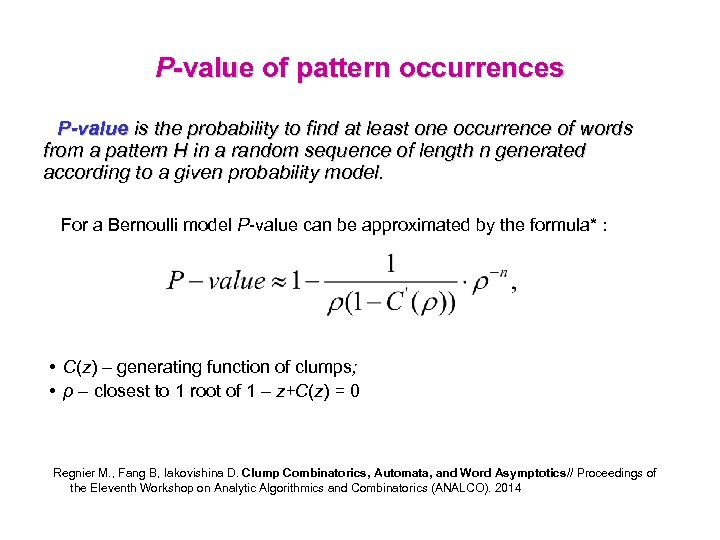P-value of pattern occurrences P-value is the probability to find at least one occurrence of words from a pattern H in a random sequence of length n generated according to a given probability model. For a Bernoulli model P-value can be approximated by the formula* : • C(z) – generating function of clumps; • ρ – closest to 1 root of 1 – z+C(z) = 0 Regnier M. , Fang B, Iakovishina D. Clump Combinatorics, Automata, and Word Asymptotics// Proceedings of the Eleventh Workshop on Analytic Algorithmics and Combinatorics (ANALCO). 2014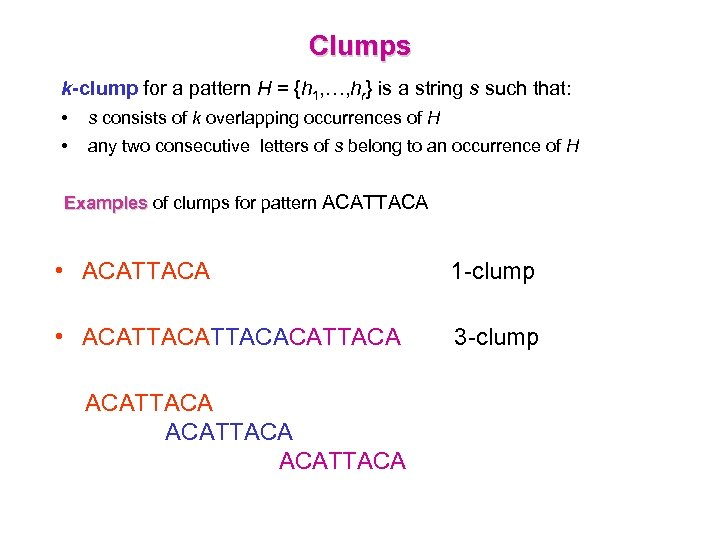Clumps k-clump for a pattern H = {h 1, …, hr} is a string s such that: • s consists of k overlapping occurrences of H • any two consecutive letters of s belong to an occurrence of H Examples of clumps for pattern ACATTACA Examples • ACATTACA 1 -clump • ACATTACACATTACA 3 -clump ACATTACA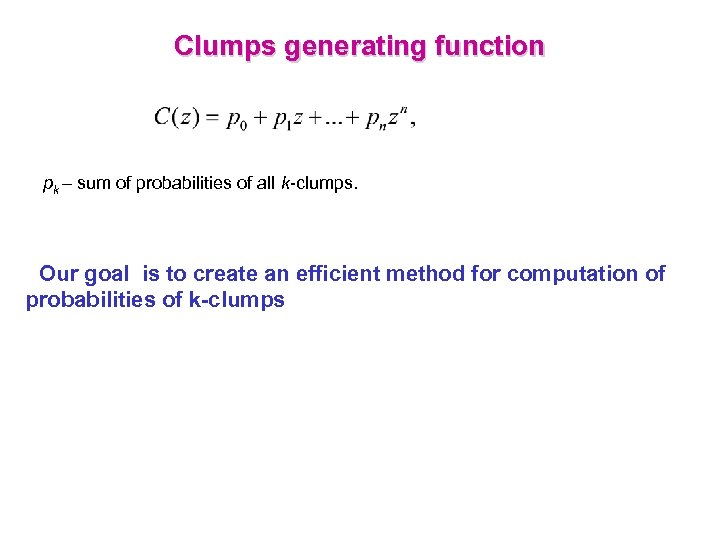Clumps generating function pk – sum of probabilities of all k-clumps. Our goal is to create an efficient method for computation of probabilities of k-clumps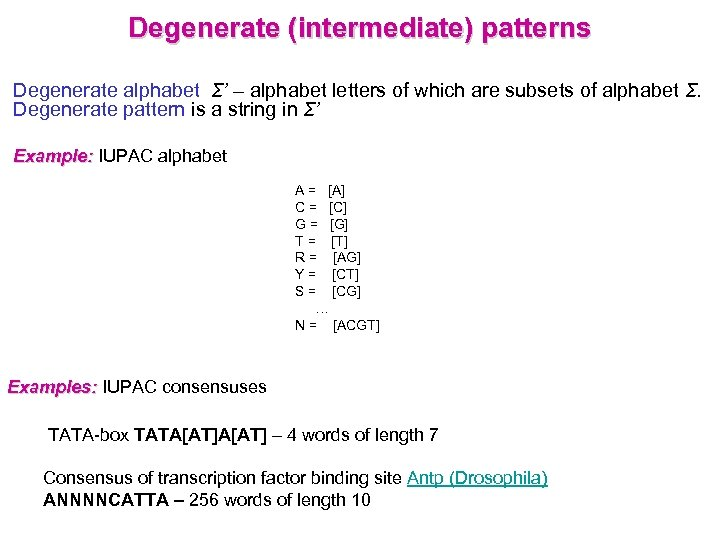Degenerate (intermediate) patterns Degenerate alphabet Σ’ – alphabet letters of which are subsets of alphabet Σ. Degenerate pattern is a string in Σ’ Example: IUPAC alphabet A = [A] C = [C] G = [G] T = [T] R = [AG] Y = [CT] S = [CG] … N = [ACGT] Examples: IUPAC consensuses ТАТА-box ТAТA[AТ] – 4 words of length 7 Consensus of transcription factor binding site Antp (Drosophila) ANNNNCATTA – 256 words of length 10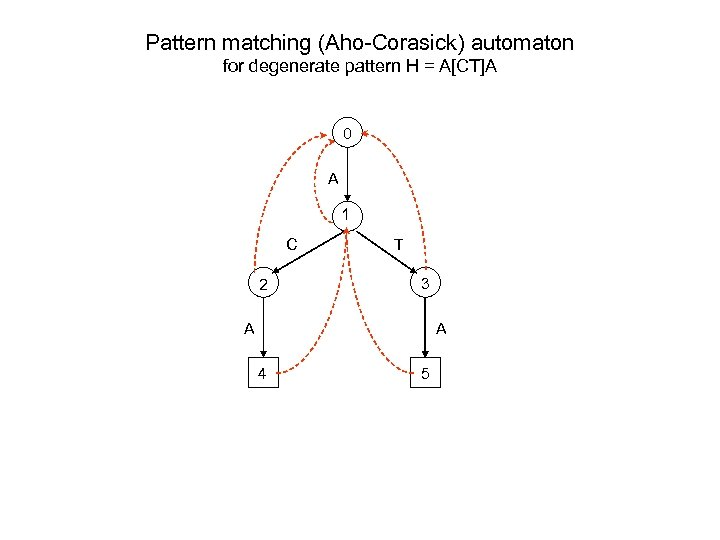Pattern matching (Aho-Corasick) automaton for degenerate pattern H = A[CT]A 0 A 1 C 2 T 3 A A 4 5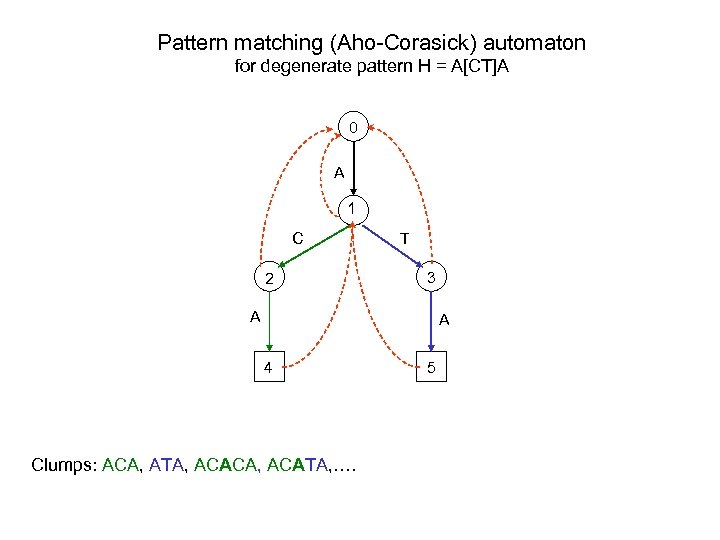Pattern matching (Aho-Corasick) automaton for degenerate pattern H = A[CT]A 0 A 1 C 2 T 3 A A 4 Clumps: ACA, ATA, ACACA, ACATA, …. 5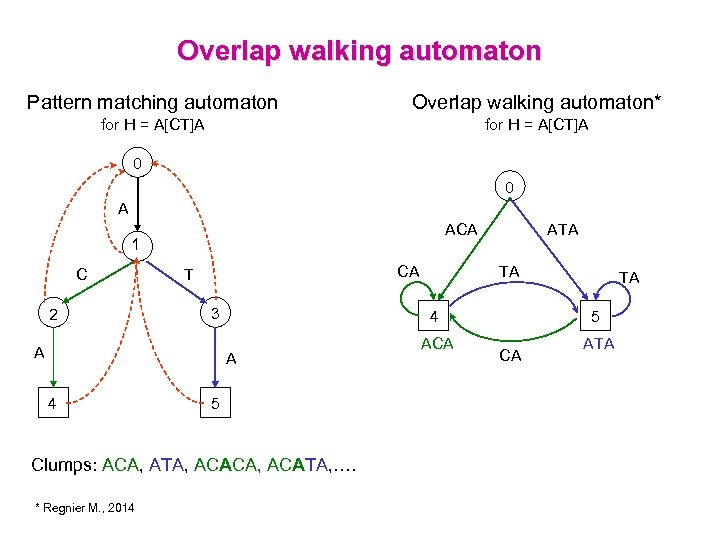Overlap walking automaton Pattern matching automaton Overlap walking automaton* for H = A[CT]A 0 0 A ACA 1 C 2 CA T 3 A 5 Clumps: ACA, ATA, ACACA, ACATA, …. * Regnier M. , 2014 TA ACA TA 5 4 ATA CA ATA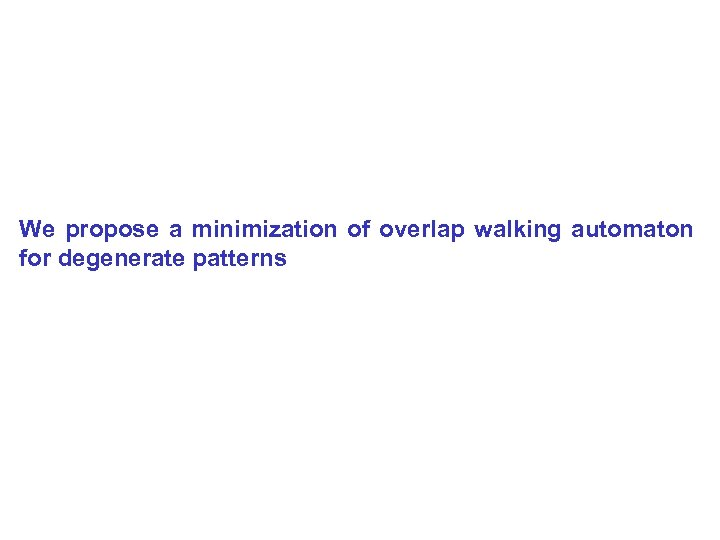We propose a minimization of overlap walking automaton for degenerate patterns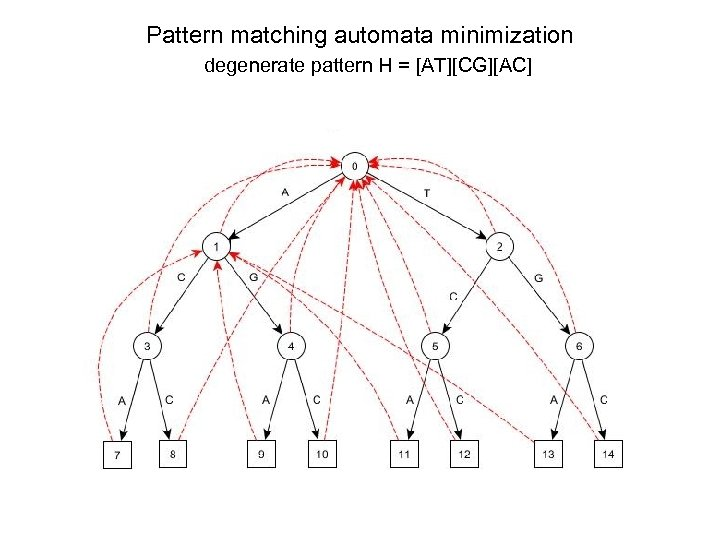Pattern matching automata minimization degenerate pattern H = [AT][CG][AC]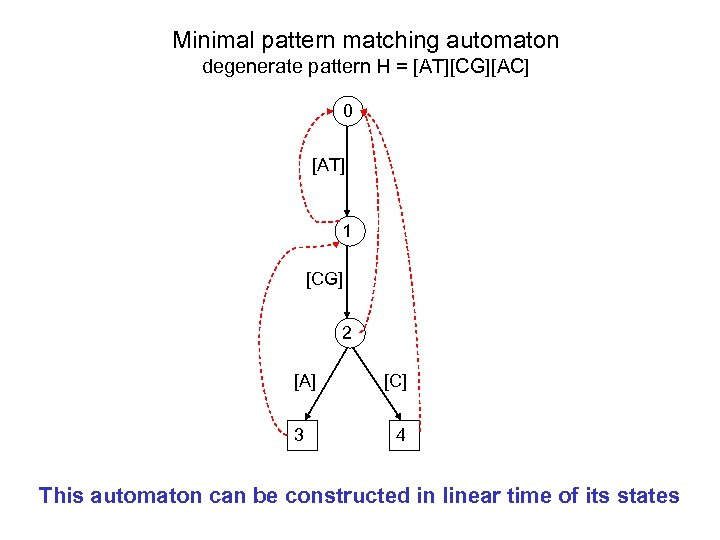Minimal pattern matching automaton degenerate pattern H = [AT][CG][AC] 0 [AT] 1 [CG] 2 [A] 3 [C] 4 This automaton can be constructed in linear time of its states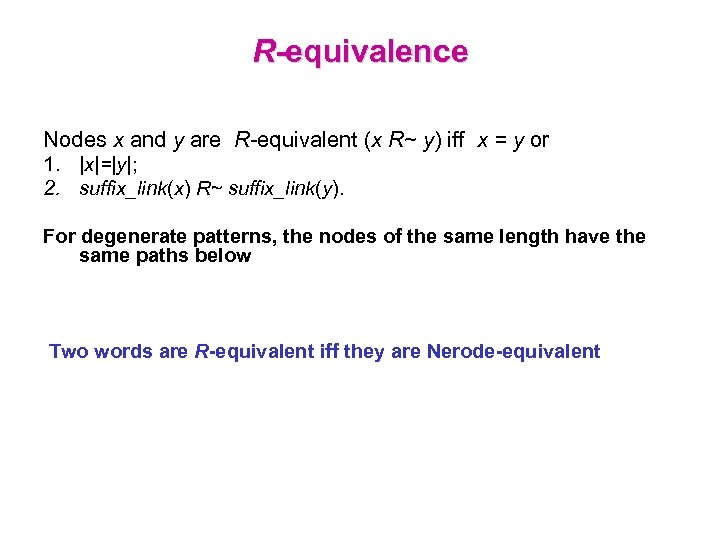R-equivalence Nodes x and y are R-equivalent (x R~ y) iff x = y or 1. |x|=|y|; 2. suffix_link(x) R~ suffix_link(y). For degenerate patterns, the nodes of the same length have the same paths below Two words are R-equivalent iff they are Nerode-equivalent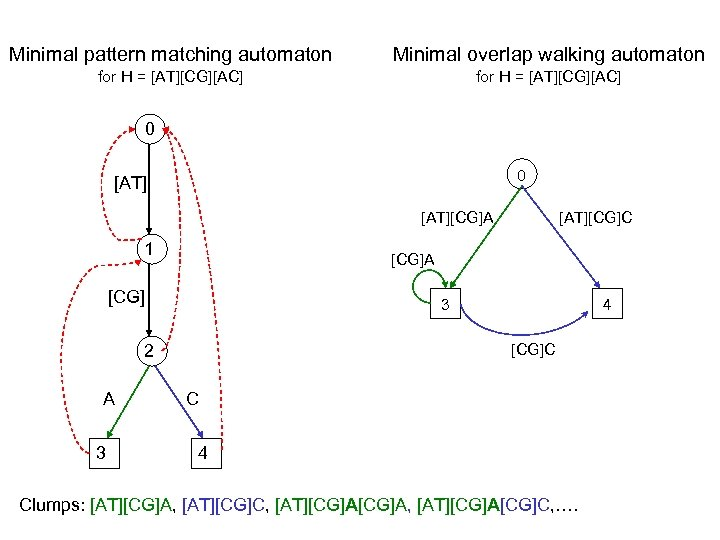Minimal pattern matching automaton Minimal overlap walking automaton for H = [AT][CG][AC] 0 0 [AT][CG]A 1 [CG]A [CG] 3 3 4 [CG]C 2 A [AT][CG]C C 4 Clumps: [AT][CG]A, [AT][CG]C, [AT][CG]A, [AT][CG]A[CG]C, ….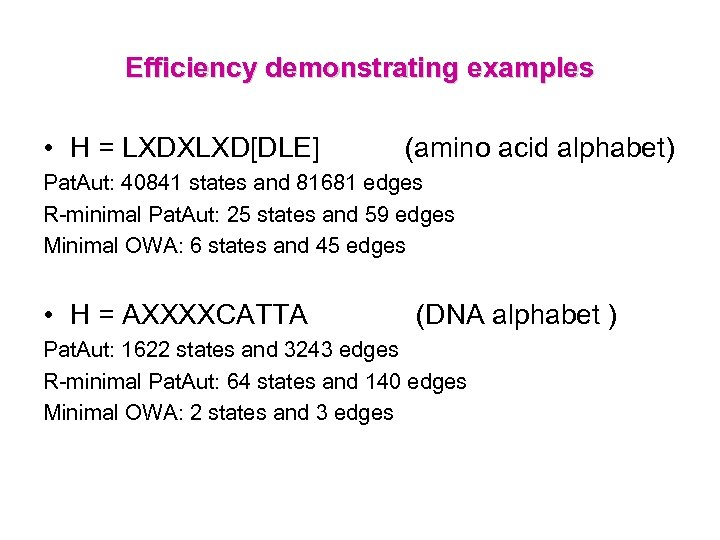Efficiency demonstrating examples • H = LXDXLXD[DLE] (amino acid alphabet) Pat. Aut: 40841 states and 81681 edges R-minimal Pat. Aut: 25 states and 59 edges Minimal OWA: 6 states and 45 edges • H = AXXXXCATTA (DNA alphabet ) Pat. Aut: 1622 states and 3243 edges R-minimal Pat. Aut: 64 states and 140 edges Minimal OWA: 2 states and 3 edgesMerci